The HPMIXED Procedure
 Computing Starting Values by EM-REML

The EM-REML algorithm (Dempster, Laird, and Rubin; 1977) iteratively alternates between an expectation step and a maximization step to maximize the restricted log likelihood. The algorithm is based on augmenting the observed data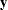with the unobservable random effects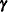, leading to a simplified form for the log likelihood. For example, if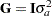then given the realized values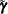of the unobservable random effects, the REML estimate of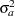satisfies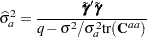This corresponds to the maximization step of EM-REML. However, the true realized valuesare unknown in practice. The expectation step of EM-REML replaces them with the conditional expected values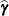of the random effects, given the observed dataand initial values for the parameters. The new estimate ofis used in turn to recalculate the conditional expected values, and the iteration is repeated until convergence.

It is well known that EM-REML is generally more robust against a poor choice of starting values than general nonlinear optimization methods such as Newton-Raphson, though it tends to converge slowly as it approaches the optimum. The Newton-Raphson method, on the other hand, converges much faster when it has a good set of starting values. The HPMIXED procedure, thus, employs a scheme that uses EM-REML initially in order to get good starting values, and after a few iterations, when the decrease in log likelihood has significantly slowed down, switching to a more general nonlinear optimization technique (by default, quasi-Newton).Previous Page | Next Page | Top of Page